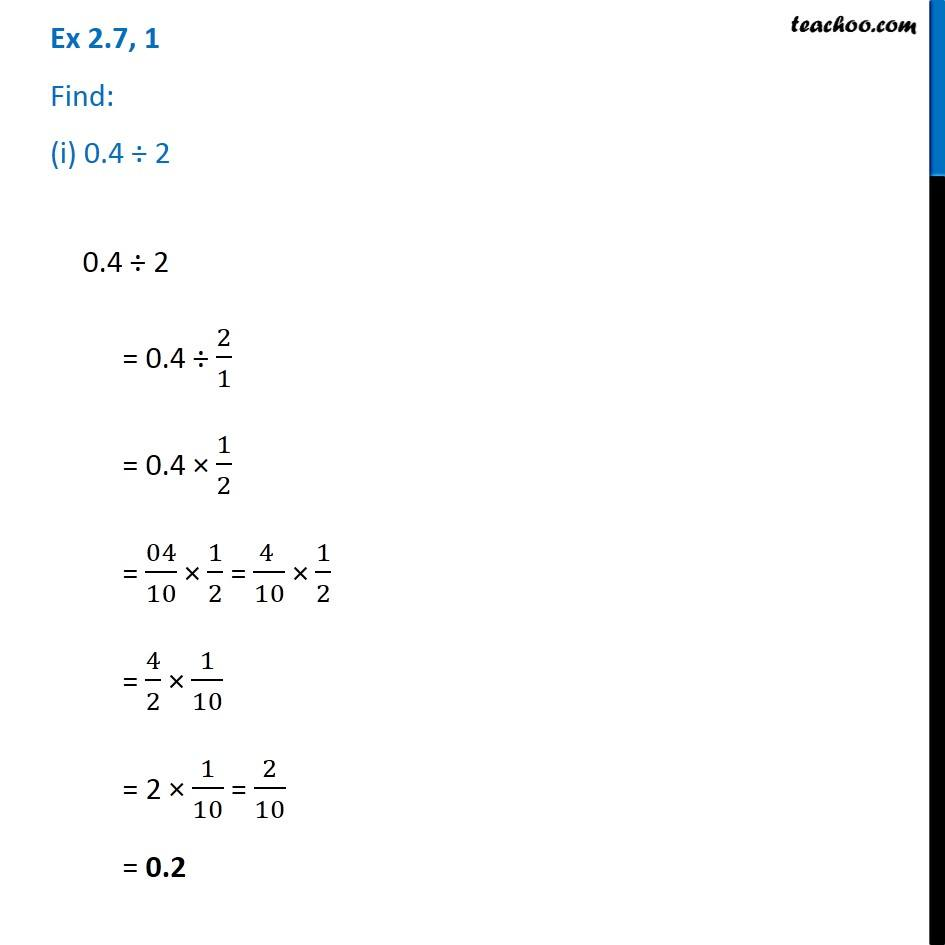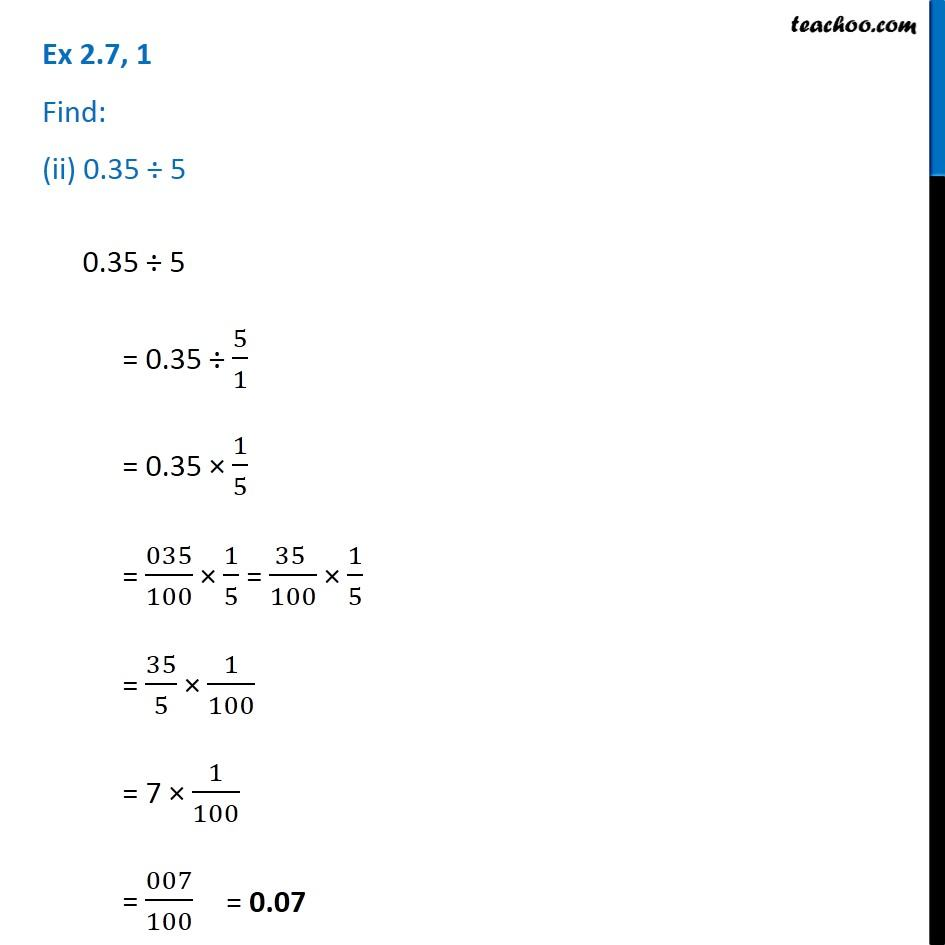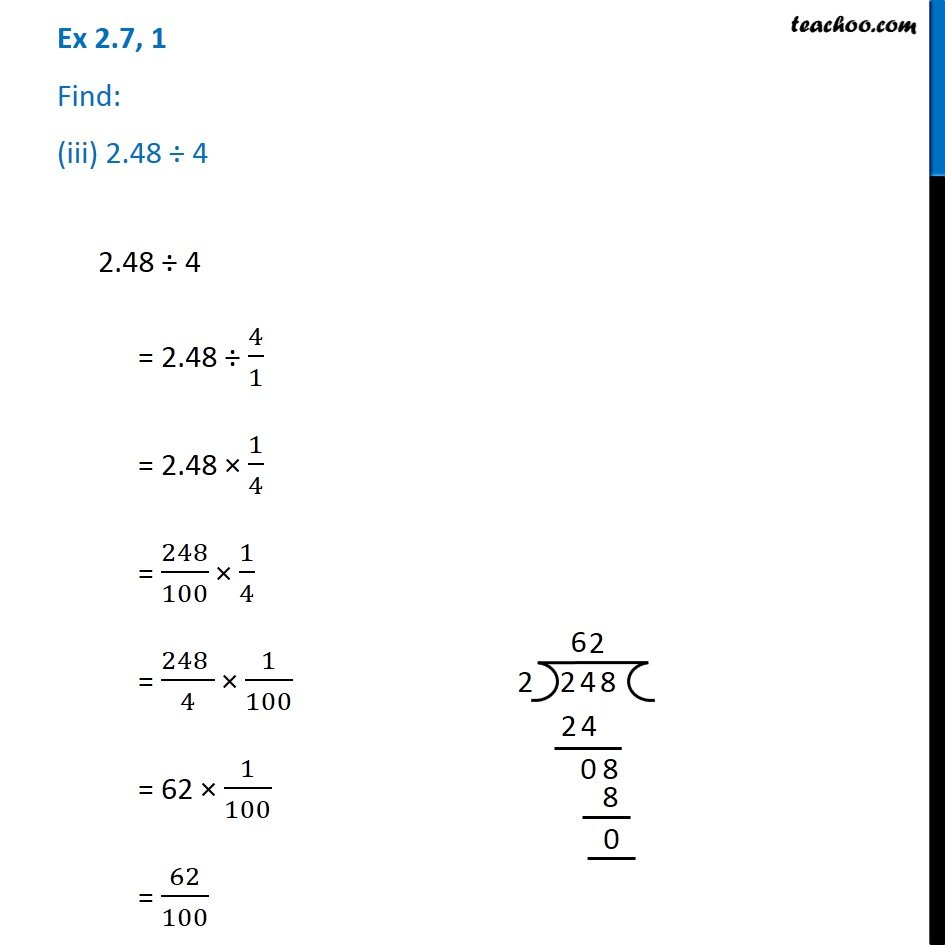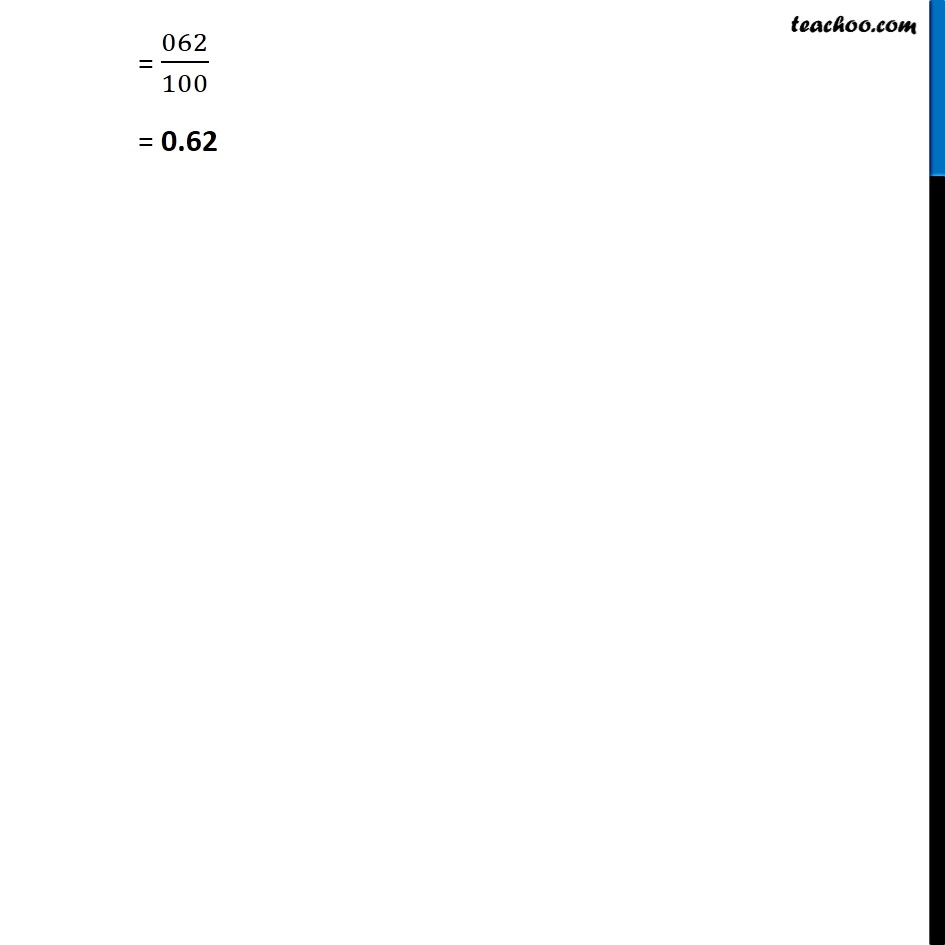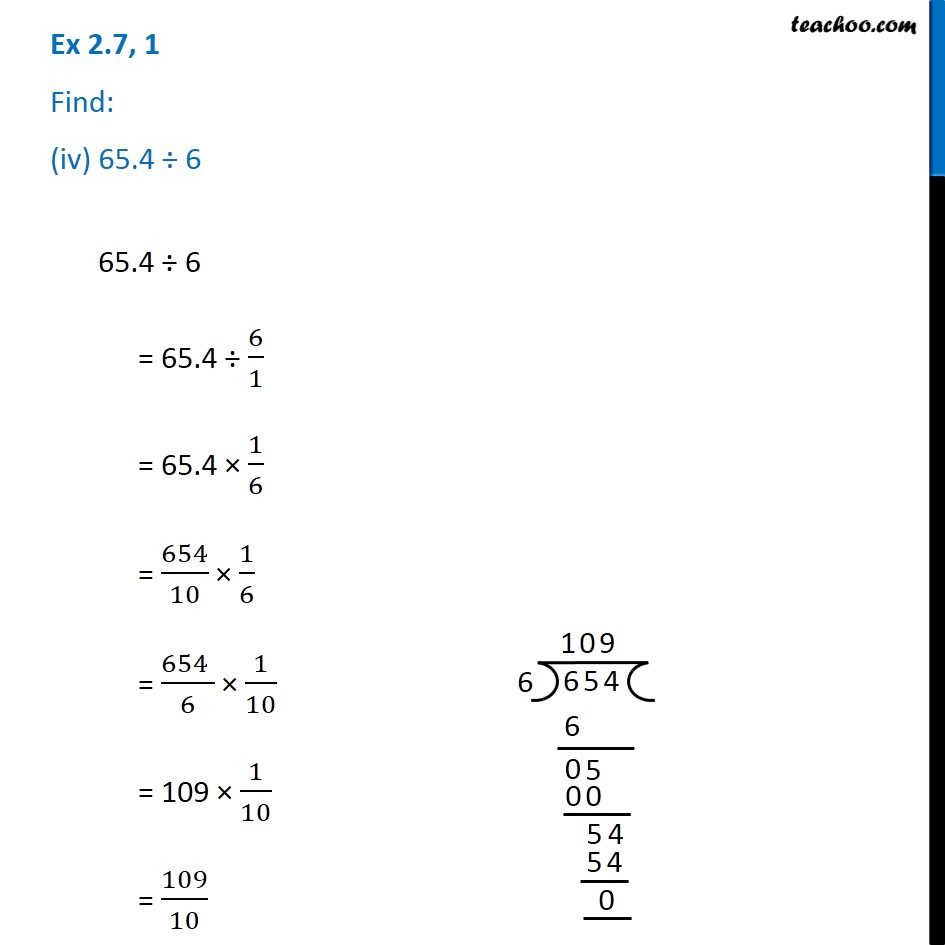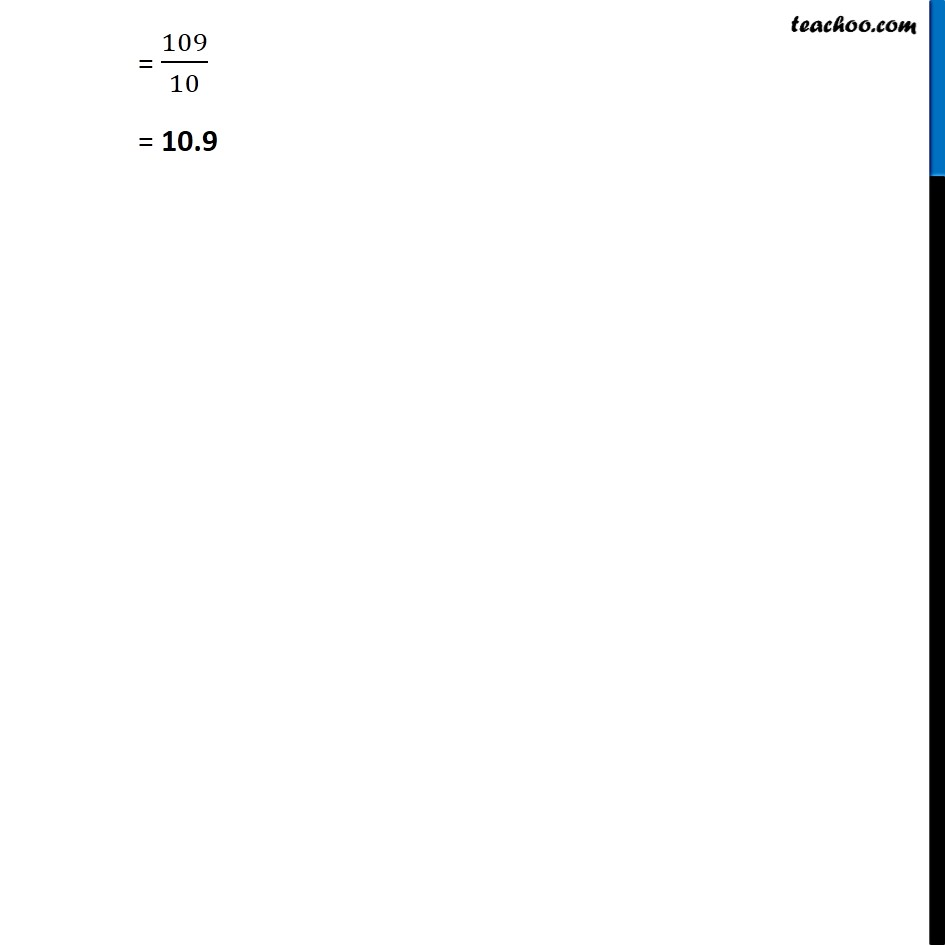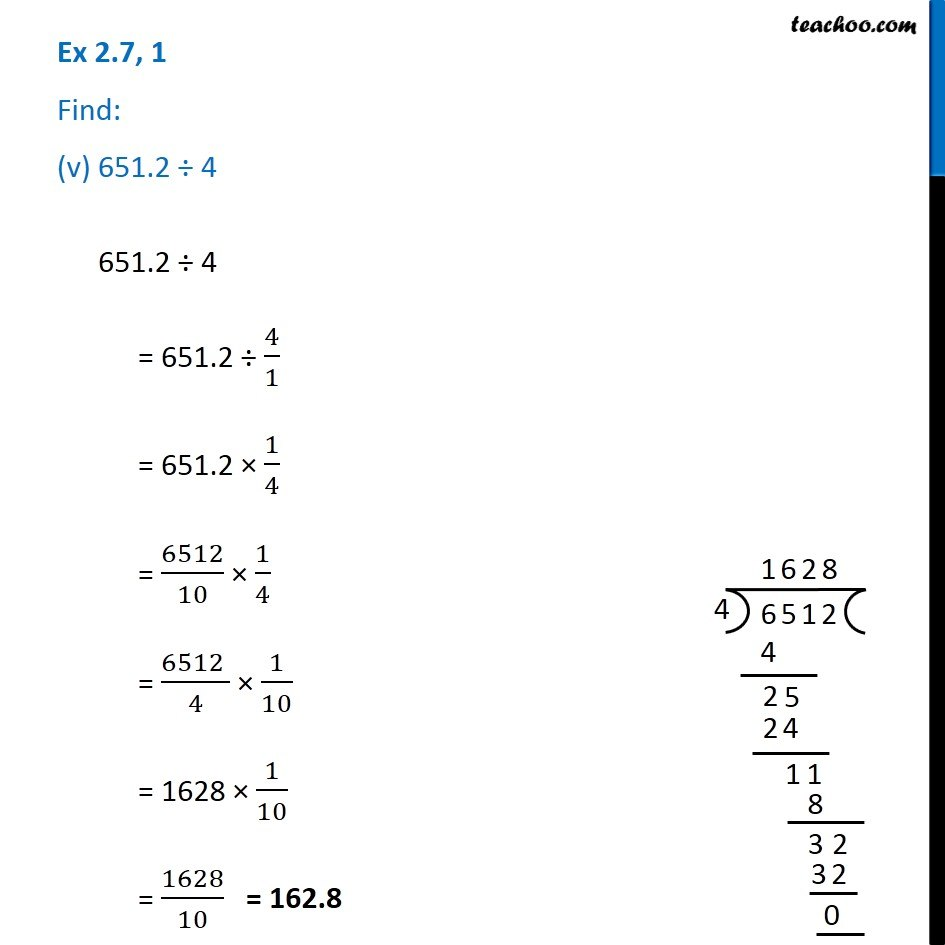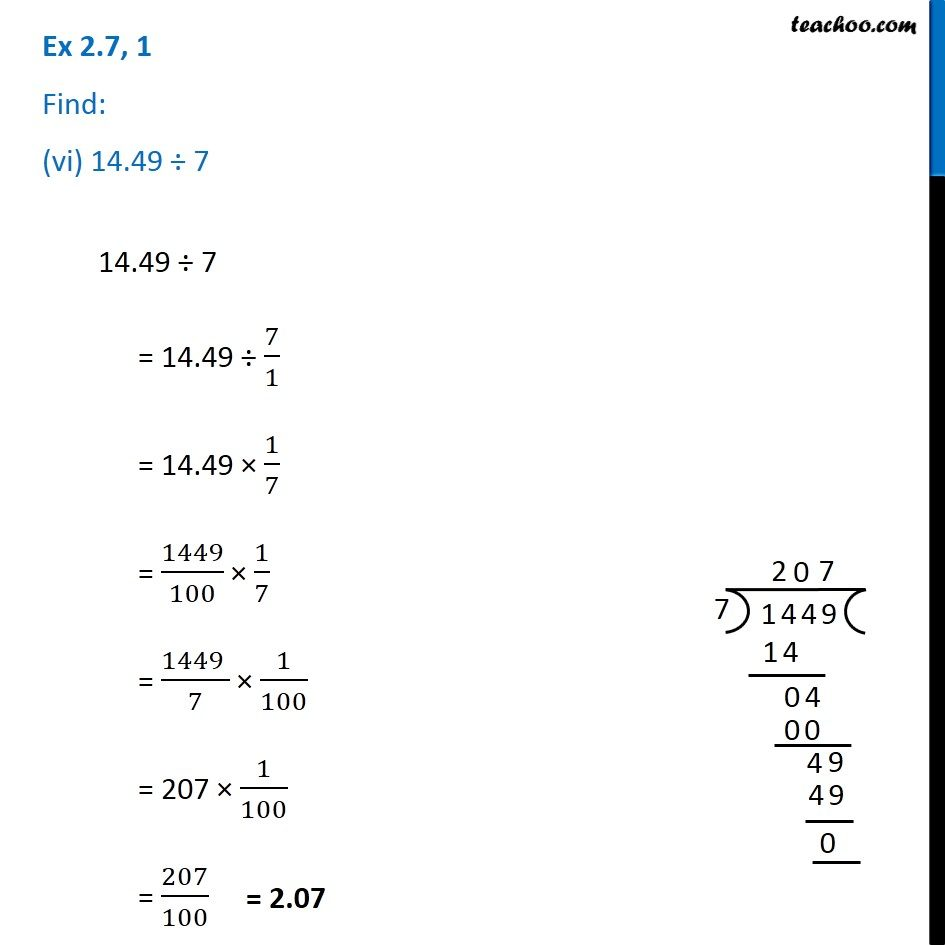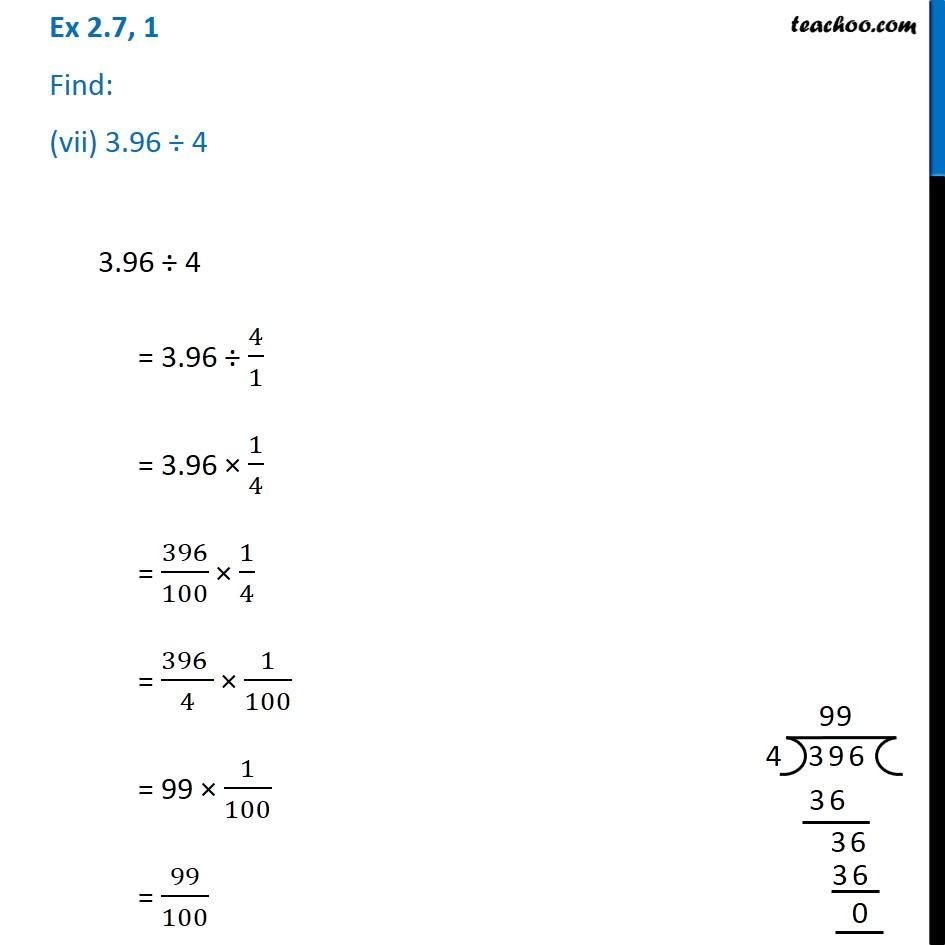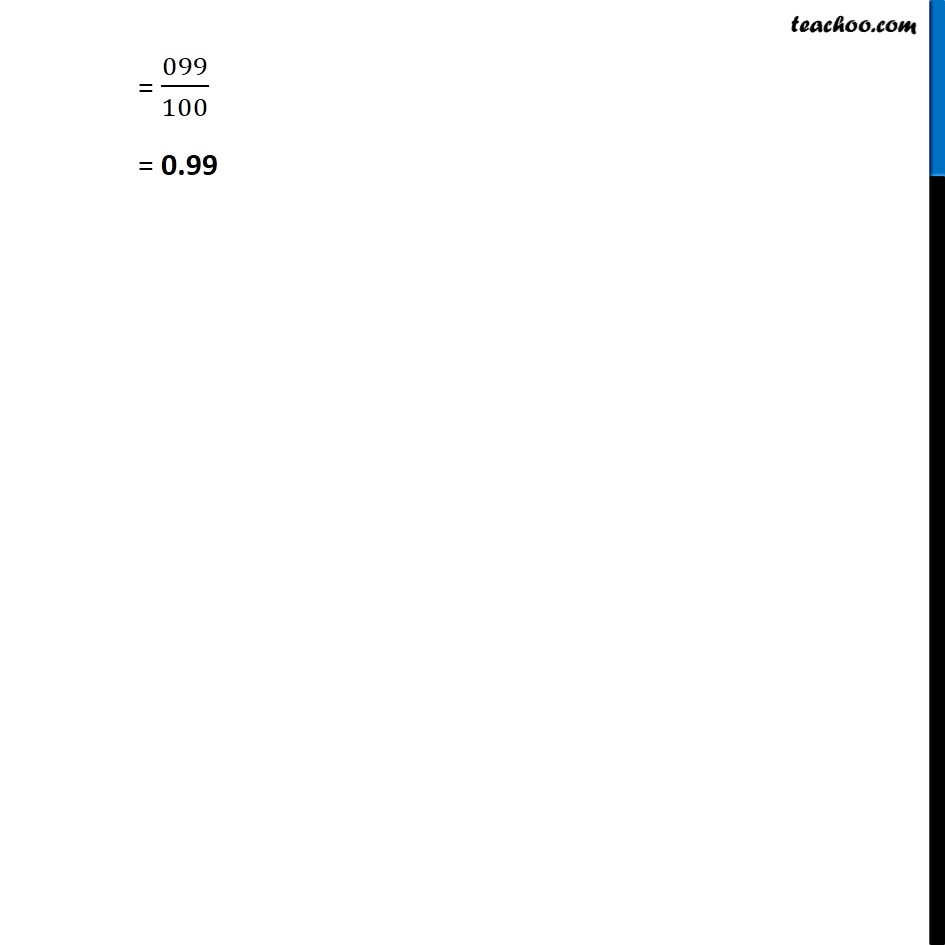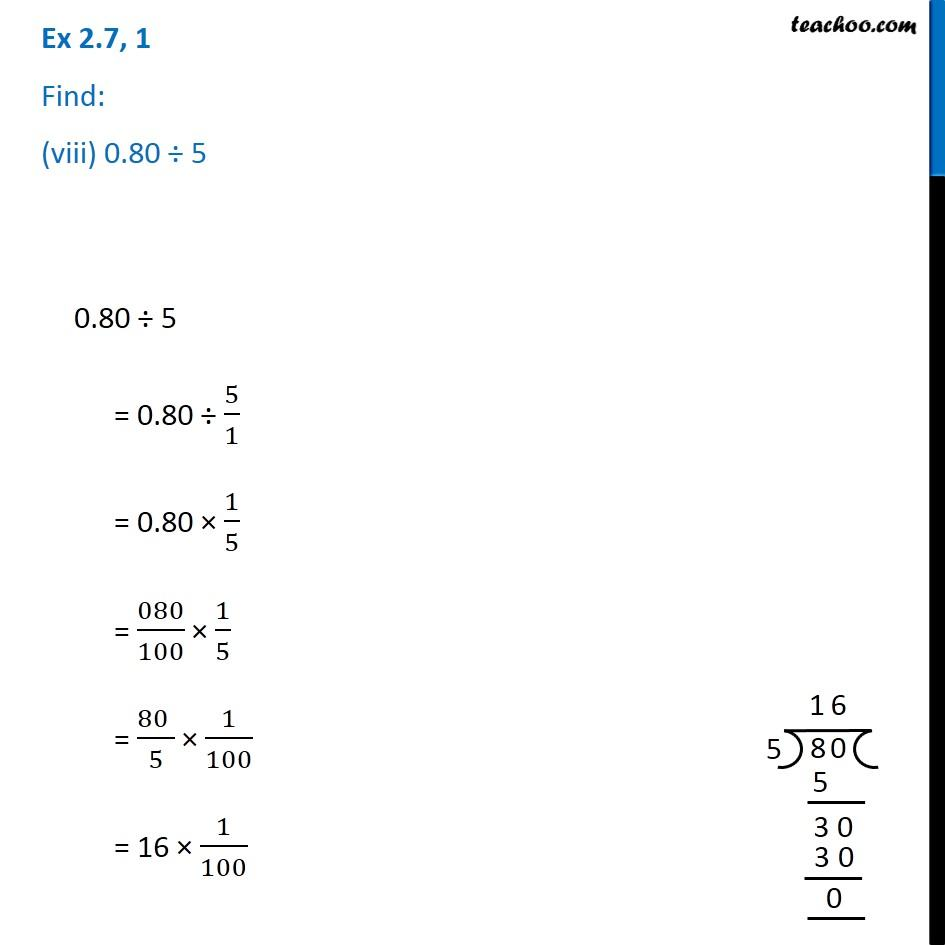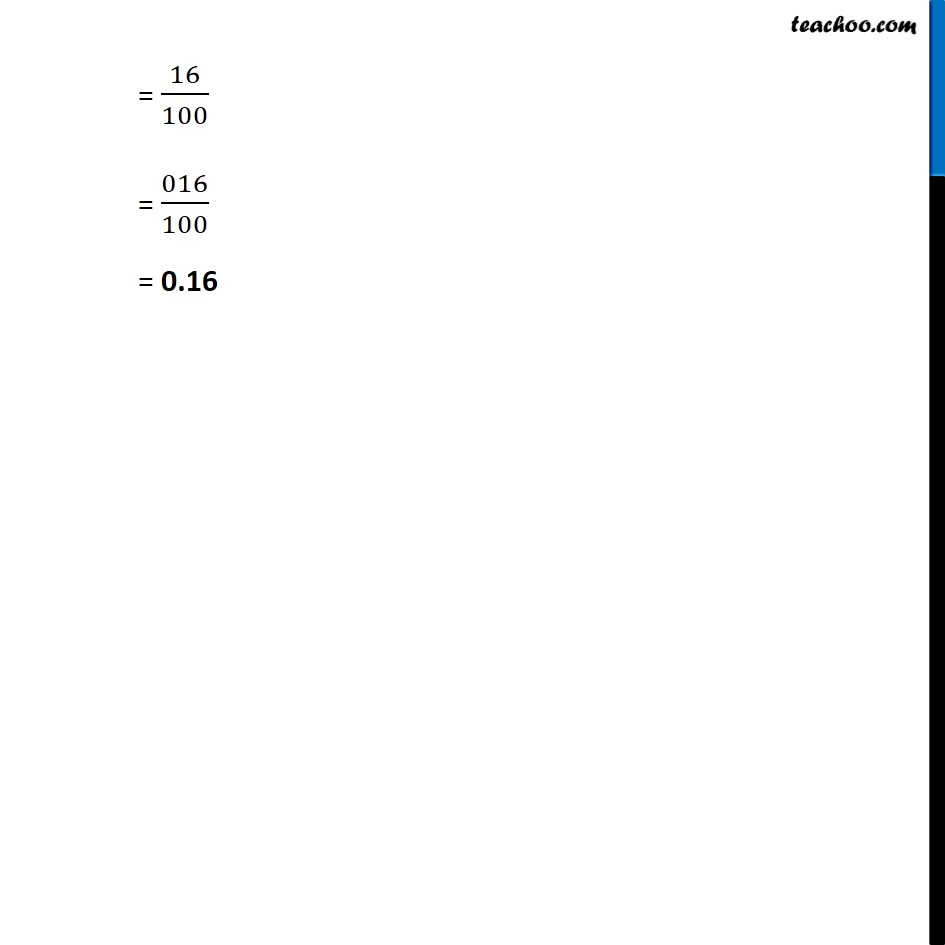1. Chapter 2 Class 7 Fractions and Decimals
2. Serial order wise
3. Ex 2.7

Transcript

Ex 2.7, 1 Find: (i) 0.4 ÷ 2 0.4 ÷ 2 = 0.4 ÷ 2/1 = 0.4 × 1/2 = 04/10 × 1/2 = (4 )/10 × 1/2 = 4/2 × 1/10 = 2 × 1/10 = 2/10 = 0.2 Ex 2.7, 1 Find: (ii) 0.35 ÷ 5 0.35 ÷ 5 = 0.35 ÷ 5/1 = 0.35 × 1/5 = 035/100 × 1/5 = (35 )/100 × 1/5 = 35/5 × 1/100 = 7 × 1/100 = 007/100Ex 2.7, 1 Find: (iii) 2.48 ÷ 42.48 ÷ 4 = 2.48 ÷ 4/1 = 2.48 × 1/4 = 248/100 × 1/4 = (248 )/4 × 1/100 = 62 × 1/100 = 62/100 = 062/100 = 0.62 Ex 2.7, 1 Find: (iv) 65.4 ÷ 665.4 ÷ 6 = 65.4 ÷ 6/1 = 65.4 × 1/6 = 654/10 × 1/6 = (654 )/6 × 1/10 = 109 × 1/10 = 109/10 = 109/10 = 10.9 Ex 2.7, 1 Find: (v) 651.2 ÷ 4 651.2 ÷ 4 = 651.2 ÷ 4/1 = 651.2 × 1/4 = 6512/10 × 1/4 = (6512 )/4 × 1/10 = 1628 × 1/10 = 1628/10 Ex 2.7, 1 Find: (vi) 14.49 ÷ 7 14.49 ÷ 7 = 14.49 ÷ 7/1 = 14.49 × 1/7 = 1449/100 × 1/7 = (1449 )/7 × 1/100 = 207 × 1/100 = 207/100 Ex 2.7, 1 Find: (vii) 3.96 ÷ 4 3.96 ÷ 4 = 3.96 ÷ 4/1 = 3.96 × 1/4 = 396/100 × 1/4 = (396 )/4 × 1/100 = 99 × 1/100 = 99/100= 099/100 = 0.99 Ex 2.7, 1 Find: (viii) 0.80 ÷ 50.80 ÷ 5 = 0.80 ÷ 5/1 = 0.80 × 1/5 = 080/100 × 1/5 = (80 )/5 × 1/100 = 16 × 1/100 Ex 2.7, 1 Find: (viii) 0.80 ÷ 50.80 ÷ 5 = 0.80 ÷ 5/1 = 0.80 × 1/5 = 080/100 × 1/5 = (80 )/5 × 1/100 = 16 × 1/100 = 16/100 = 016/100 = 0.16

Ex 2.7

About the AuthorDavneet Singh
Davneet Singh is a graduate from Indian Institute of Technology, Kanpur. He has been teaching from the past 10 years. He provides courses for Maths and Science at Teachoo.